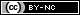# Week 5 - VectorsLoading previews...
 Vectors HTMLViewVectors
1 file in this resource
Summary: This unit provides an introduction to vectors. We begin by defining what is meant by the term vector and describe how we distinguish vectors from scalars. The main properties of vectors are presented and the concept of a position vector is introduced. We then look at operations on vectors such as addition, subtraction and scalar multiplication both algebraically and graphically. The idea of a unit vector is introduced and we look at how to express the position vector of a point, in two and three dimensions, in Cartesian components using the standard unit vectors in the directions of the coordinate axes. The unit closes with a look at how to calculate the scalar (dot) product of two vectors. DR Calum MacDonald MR Leonard Scott Academic > School of Computing, Engineering and Built Environment Copyright © Glasgow Caledonian University Vectors World MR Joseph Mills 17 Feb 2017 11:50 17 Feb 2017 11:50 https://edshare.gcu.ac.uk/id/eprint/2299View Item